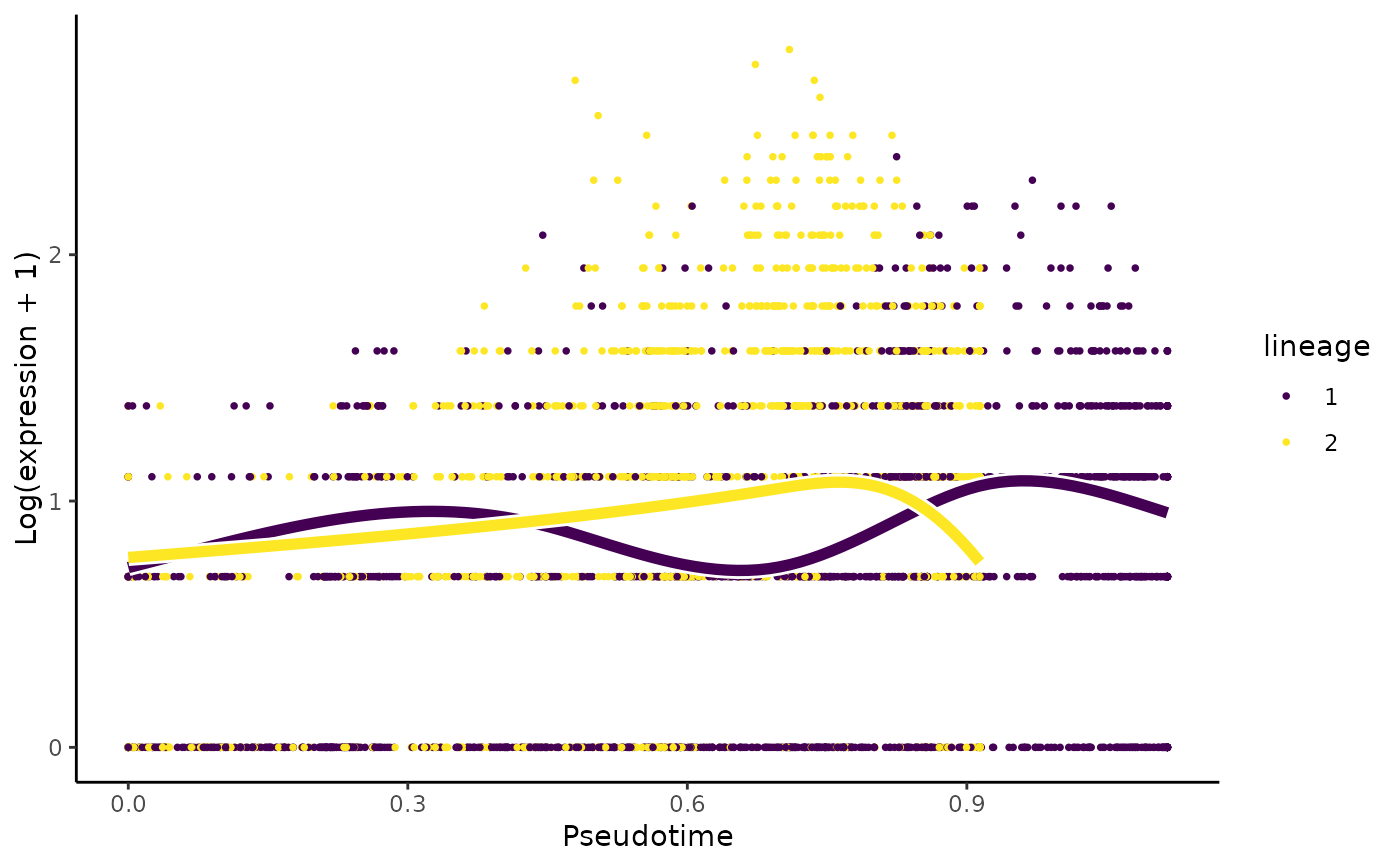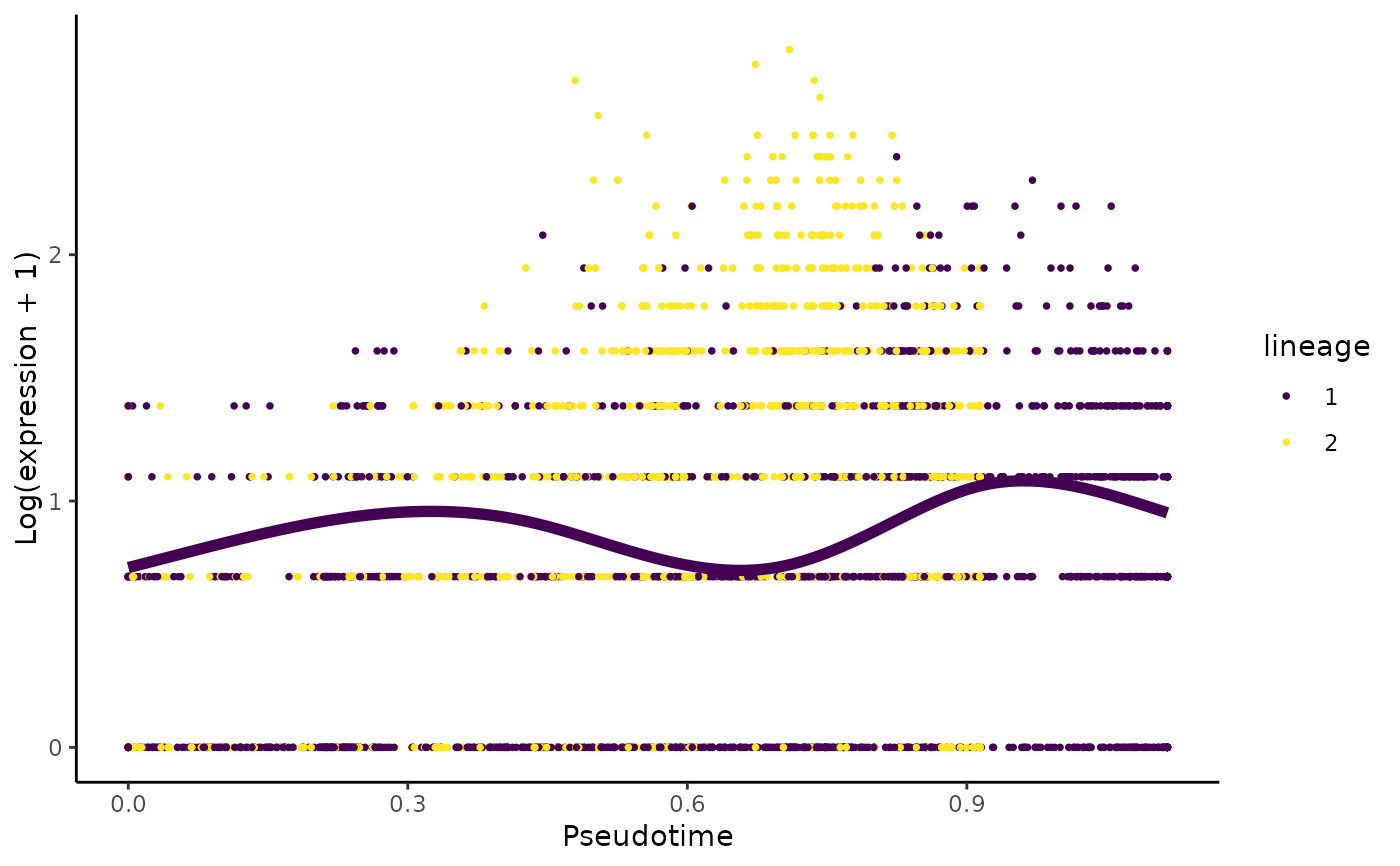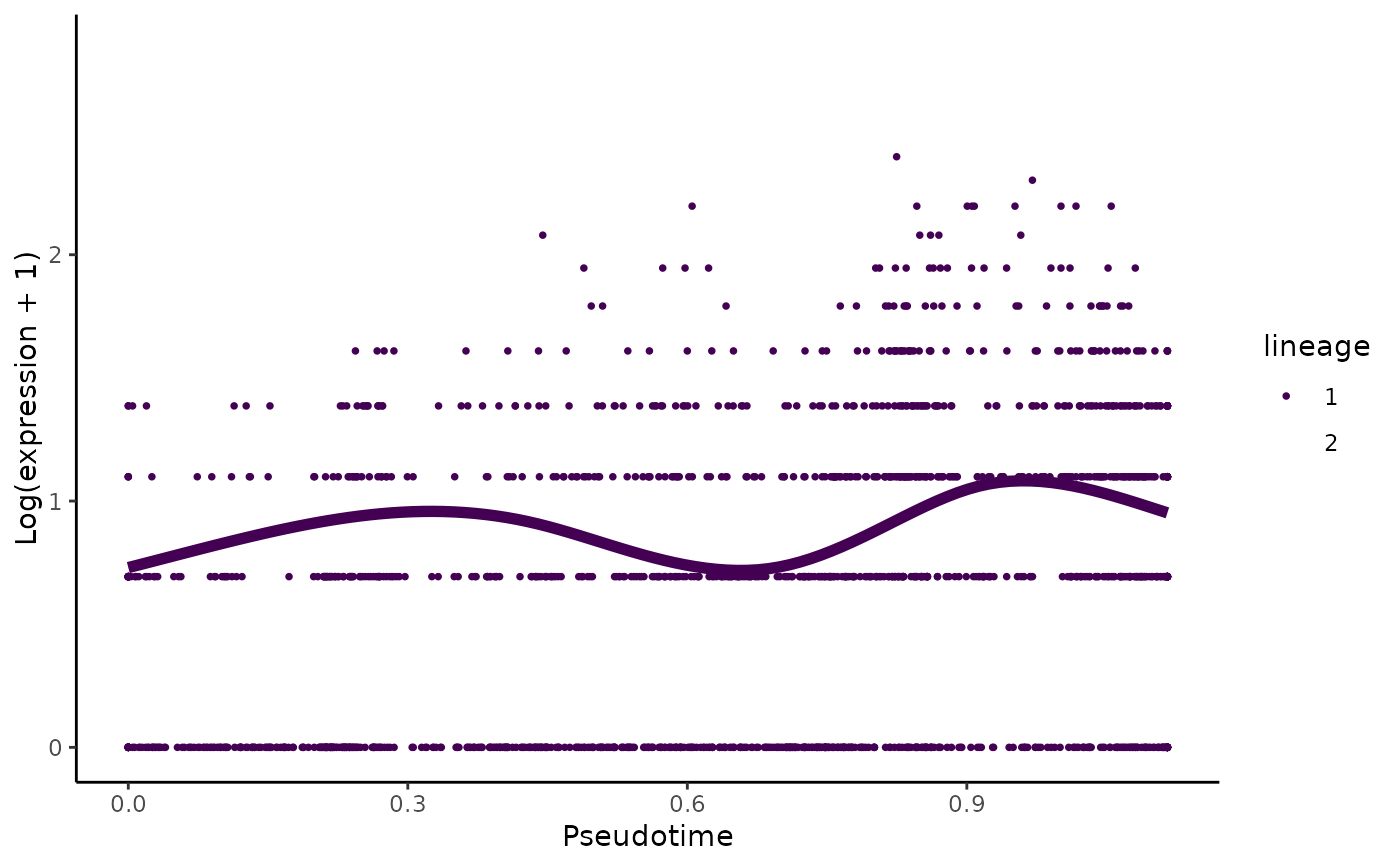Plot the smoothers estimated by tradeSeq.

plotSmoothers(models, ...)

# S4 method for gam
plotSmoothers(
models,
nPoints = 100,
lwd = 2,
size = 2/3,
xlab = "Pseudotime",
ylab = "Log(expression + 1)",
border = TRUE,
alpha = 1,
sample = 1
)

# S4 method for SingleCellExperiment
plotSmoothers(
models,
counts,
gene,
nPoints = 100,
lwd = 2,
size = 2/3,
xlab = "Pseudotime",
ylab = "Log(expression + 1)",
border = TRUE,
alpha = 1,
sample = 1,
pointCol = NULL,
curvesCols = NULL,
plotLineages = TRUE
)

## Arguments

models Either the SingleCellExperiment object obtained after running fitGAM, or the specific GAM model for the corresponding gene, if working with the list output of tradeSeq. parameters including: The number of points used to extrapolate the fit. Defaults to 100. Line width of the smoother. Passed to geom_line. Character expansion of the data points. Passed to geom_point. x-axis label. Passed to labs. y-axis label. Passed to labs. Logical: should a white border be drawn around the mean smoother. Numeric between 0 and 1, determines the transparency of data points, see scale_color_viridis_d. Numeric between 0 and 1, use to subsample the cells when there are too many so that it can plot faster. The matrix of gene expression counts. Gene name or row in count matrix of gene to plot. Plotting colors for each cell. Can be either character vector of length 1, denoting a variable in the colData(models) to color cells by, or a vector of length equal to the number of cells. Plotting colors for each curve Should be a list of colors of the exact same length as the number of curves, i.e. the number of lineages (if there is no conditions) or the number of lineages by the number of conditions. In the second case, the colors are grouped by condition (lineage 1 - condition 1, lineage 1 - condition 2,...). Logical, should the mean smoothers for each lineage be plotted?

## Value

A ggplot object

## Examples

set.seed(8)
counts <- as.matrix(countMatrix)
sce <- fitGAM(counts = counts,
sds = crv,
nknots = 5)
plotSmoothers(sce, counts, rownames(counts))# Show only first lineage curve
curvesCols <- c("#440154FF", "transparent")
plotSmoothers(sce, counts, rownames(counts), curvesCols = curvesCols,
border = FALSE)# Show only first curve and cells assigned to first lineage
plotSmoothers(sce, counts, rownames(counts), curvesCols = curvesCols,
border = FALSE) +
ggplot2::scale_color_manual(values = curvesCols)
#> Scale for 'colour' is already present. Adding another scale for 'colour',
#> which will replace the existing scale.